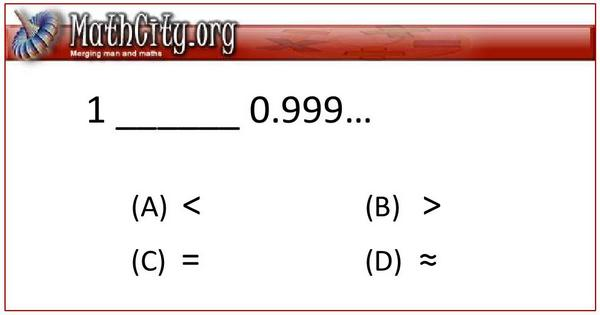Is it difficult to answer? 1−−−0.999…This is a simple and common question asked to students, who have passed higher secondary school certificate. Most of the student unable to give the correct answer. Well, we cannot predict the exact reason but one simple reason seems to be a lack of logical reasoning. For example, if we wish to round off $0.999\ldots$, then we arrived, it cannot be 0.9 or 0.99 or 0.999 or any other.

Let we wish to rounding it off to three decimals. Then the 9 at third place become 10, something like, 0.99(10). So we have to add the 1 to a 9 in the left side of (10). This gives us 0.9(10)0. Again by the similar arguments, we arrived at 0.(10)00. This lead us to 1.000, which is actually 1. Okay, that a good ideas, but what about the complete proof. There are many proofs of this fact. One is by using geometric series but we are giving here simple algebraic proof.

Consider $x=0.999\ldots$, then $10x=9.99\ldots$.

This gives us $10x=9+0.999\ldots$, this implies $10x=9+x$.

This leads to $9x=9$, which gives us $x=1$.

• dyk/4